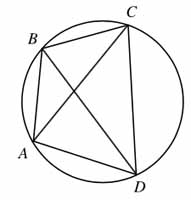# Ptolemy's theoremFor a cyclic quadrilateral, the sum of the products of the two pairs of opposite sides equals the product of the diagonals.

$AB \times CD + BC \times DA = AC \times BD$.

If $ABCD$ is a rectangle, this reduces to Pythagoras's theorem.